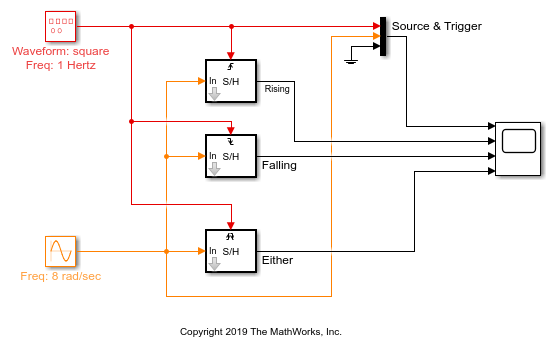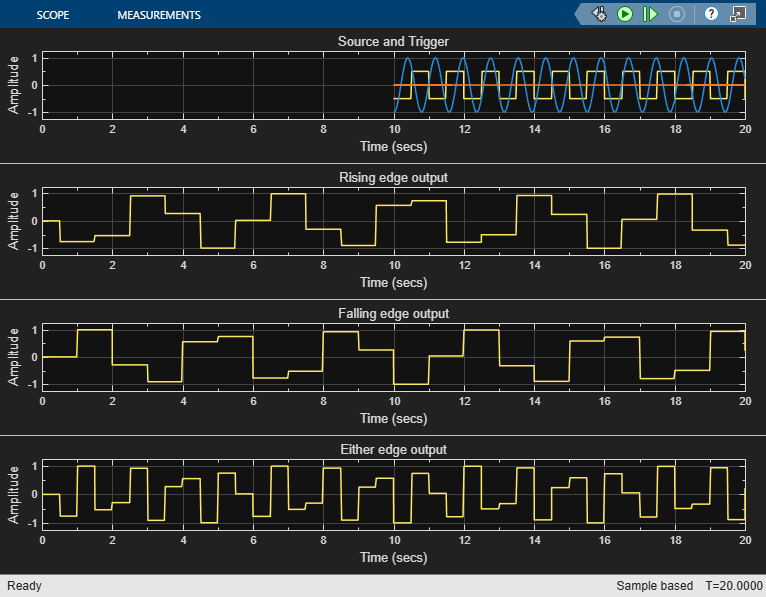# Sample and Hold a Signal

Sample an input signal when a trigger event occurs and hold the value until the next trigger event using the Sample and Hold block. The trigger event can be one of the following:

• Rising edge - Negative value or zero to a positive value.

• Falling edge - Positive value or zero to a negative value.

• Either edge - Negative value or zero to a positive value and positive value or zero to a negative value.

### Open the Model

The model contains three Sample and Hold blocks which accept the three type of trigger events. The input signal is a continuous-time sine wave with an amplitude of 1 and a frequency of 8 rad/sec. The trigger signal is a square wave with an amplitude of 0.5 and a frequency of 1 Hz.### Run the Model

When you run the model, the Time Scope block shows the source and trigger signals on the first plot. You can see the three sample and hold outputs on the three remaining plots.The Initial condition parameter in all the three Sample and Hold blocks is set to 0. Hence, the three output plots start at 0 value. The first trigger is a rising edge that happens at 0.5 seconds. The first and third outputs respond to this trigger by dropping to the value of the input sine wave at that point in time. This input value is held until the next respective trigger event occurs. The second output plot responds to the first falling edge that occurs at 1 second. At 1 second, the second output plot jumps to 1, which is the value of the sine wave at that point in time. This value is held until 2 seconds when the next falling edge event occurs. The output of the second plot then drops down to the value of the sine wave at that point in time. This value is held until the next trigger event occurs.